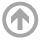Mathematics Department

# Basic Notions Seminars

## Spring 2019

All talks are from 1200--1300 in the Seminar room, unless otherwise specified.

The Basic Notions seminar features ideas which can be viewed as fundamental to some area of mathematics, or fundamental to a connection between areas of mathematics, or fundamental to an application of mathematics. The definition of "mathematics", "connection", and "application", is broad in this context. Perhaps "Fundamental Notions" is the correct synonymous title. Talks are aimed at a "graduate-level", though frequently the "basicnotion in the talk is an example of, or hints at, deep mathematical content.

• Apr
23
• TBA
Nick Wood
USNA Math
Time: 12:00 PM
• Apr
16
• Basic Notions of Philosophy of Math (working title)
Darren Creutz
USNA Math
Time: 12:00 PM
• Apr
09
• Representations of Operator Algebras and the Gelfand-Naimark-Segal Theorem
Mitch Baker
USNA Math
Time: 12:00 PM
• Mar
26
• Binomial Proofs and the Area Principle
Will Traves
USNA Math
Time: 12:00 PM

#### View Abstract

I'll discuss some elementary geometric ideas which were new to me when I first heard about them several years ago. In particular, I'll explain the role that determinants play in plane geometry and how these ideas lead to an elegant proof technique. Along the way, we'll see why determinants deserve their name, encounter the Fundamental Theorem of Invariant Theory, and learn an important application of Cramer's rule.
• Mar
19
• Data-Driven Methods for Prediction and Control of Complex Systems
Evelyn Lunasin
USNA Math
Time: 12:00 PM

#### View Abstract

In the first part of the talk I will discuss a few examples of how one integrates data into PDE models in order to accurately predict and control complex systems. In the second part of the talk I will give an overview of an emerging data driven modeling strategy which utilizes classical tools from linear algebra – the Singular Value Decomposition (SVD). Considered as the most impactful theorem in data science, SVD is an essential starting point to characterize complex fluid flows purely from collected measured data and with no governing equations needed.
• Jan
22
• How discontinuous can an integrable function be? -- An intro to measure theory as the natural extension of calculus.
Darren Creutz
USNA Math
Time: 12:00 PM

#### View Abstract

One of the few problems Riemann could not solve was a question about his own integrals: if f(x) is a bounded function on [a,b], when does the integral from a to b of f(x) dx exist (when are the lower and upper sums equal)? If f is continuous then the integral exists, and indeed f can have jump discontinuities. But if f is discontinuous at every point on a subinterval then the integral won't exist, so where is the line? Can an integrable function have infinitely many discontinuities? Could it be discontinuous on something as large as a Cantor set? I will answer these questions by presenting Lebesgue's notion of measurable sets, developed, initially, precisely to answer Riemann's question. No background beyond calculus will be assumed. If you haven't seen measure theory before, or it's just been a very long time, and you'd be interested in knowing what us analysts are doing, please join.

#### ARCHIVES